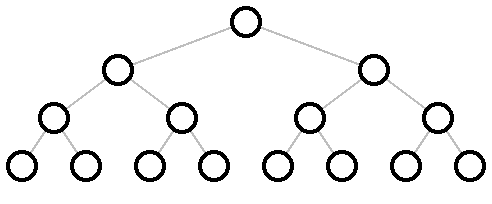### 14Rendering

 parameter(dc-for-text-size) → (or/c #f (is-a?/c dc<%>)) (dc-for-text-size dc) → void? dc : (or/c #f (is-a?/c dc<%>))
A parameter that is used to determine the bounding box of picts created with text.

The drawing context installed in this parameter need not be the same as the ultimate drawing context, but it should measure text in the same way. Under normal circumstances, font metrics are the same for all drawing contexts, so the default value of dc-for-text-size is a bitmap-dc% that draws to a 1-by-1 bitmap.

 parameter → (list/c (>=/c 0) (>=/c 0) (>=/c 0) (>=/c 0)) (convert-bounds-padding padding) → void? padding : (list/c (>=/c 0) (>=/c 0) (>=/c 0) (>=/c 0))
A parameter that determines an amount of padding added to each edge of a pict when converting to a format like 'png@2x-bytes+bounds8 (see file/convertible). The default value of the parameter is '(3 3 3 3), which adds three pixels to each edge to accommodate a small amount of drawing outside the pict’s bounding box.

Added in version 1.2 of package pict-lib.

 procedure(draw-pict pict dc x y) → void? pict : pict-convertible? dc : (is-a?/c dc<%>) x : real? y : real?
Draws pict to dc, with its top-left corner at offset (x, y).

procedure

 (pict->bitmap pict [ smoothing #:make-bitmap make-a-bitmap]) → (is-a?/c bitmap%)
pict : pict-convertible?
smoothing : (or/c 'unsmoothed 'smoothed 'aligned) = 'aligned
make-a-bitmap :
 (exact-positive-integer? exact-positive-integer? . -> . (is-a?/c bitmap%))
= make-bitmap
Returns a bitmap% with pict drawn on it in the top-left corner (0, 0). Drawing the pict into the bitmap uses the smoothing mode smoothing; see set-smoothing for more information on smoothing modes.

The make-a-bitmap function is used to create the bitmap, giving it the size of pict rounded up to integer dimensions. The default function, make-bitmap, creates a bitmap with an alpha channel. Supply make-screen-bitmap, instead, to create a bitmap that is consistent with drawing directly to the screen.

Changed in version 1.7 of package pict-lib: Added the #:make-bitmap argument.

 procedure(pict->argb-pixels pict [smoothing]) → bytes? pict : pict-convertible? smoothing : (or/c 'unsmoothed 'smoothed 'aligned) = 'aligned
Returns the bytes? with the pixels corresponding the bitmap that pict->bitmap returns. Each pixel has four bytes in the result: the alpha, red, green, and blue components.

Examples:
 > (pict->argb-pixels (filled-rectangle 1 1))

#"\377\0\0\0"

 > (pict->argb-pixels (colorize (filled-rectangle 1 1) "red"))

#"\377\377\0\0"

Added in version 1.1 of package pict-lib.

 procedure(argb-pixels->pict bytes width) → pict? bytes : bytes? width : exact-nonnegative-integer?
Constructs a pict from bytes with the width width. Each pixel in the resulting pict corresponds to four entries in bytes: the alpha value, and the red, green, and blue values.

Example:
 > (let ([b (make-bytes (* 40 40 4) 255)]) (for ([x (in-range (bytes-length b))]) ; when in one of two vertical bands (10-20 & 30-40) (when (or (<= 10 (modulo (quotient x 4) 40) 20) (<= 30 (modulo (quotient x 4) 40) 40)) ; change the red and green fields of the pixel (when (= 1 (modulo x 4)) (bytes-set! b x 0)) (when (= 2 (modulo x 4)) (bytes-set! b x 150)))) (argb-pixels->pict b 40))Added in version 1.1 of package pict-lib.

 procedure(make-pict-drawer pict) → ((is-a?/c dc<%>) real? real? . -> . void?) pict : pict-convertible?
Generates a pict-drawer procedure for multiple renderings of pict. Using the generated procedure can be faster than repeated calls to draw-pict.

procedure

 (show-pict pict [ w h] #:frame-x frame-x #:frame-y frame-y #:frame-style frame-style) → void?
pict : pict-convertible?
w : (or/c #f exact-nonnegative-integer?) = #f
h : (or/c #f exact-nonnegative-integer?) = #f
frame-x : (or/c (integer-in -10000 10000) #f)
frame-y : (or/c (integer-in -10000 10000) #f)
frame-style :